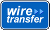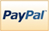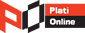Categories

View all categories

Model and analyze financial and economic systems using statistical methods

Provides functions for modeling economic data. You can select and calibrate economic models for simulation and forecasting. Time series capabilities include univariate ARMAX/GARCH composite models with several GARCH variants, multivariate VARMAX models, and cointegration analysis. The toolbox provides Monte Carlo methods for simulating systems of linear and nonlinear stochastic differential equations and a variety of diagnostics for model selection, including hypothesis, unit root, and stationarity tests.

### NOTE:

 Full Product 1.200,00 EUR
MTHWRKS-ECTO
Computational Finance

Analyze financial data and develop financial models

Provides functions for mathematical modeling and statistical analysis of financial data.  You can optimize portfolios of financial instruments, optionally taking into account turnover and transaction costs.  The toolbox enables you to estimate risk, analyze interest rate levels, price equity and interest rate derivatives, and measure investment performance.  Time series analysis functions and an app let you perform transformations or regressions with missing data and convert between different trading calendars and day-count conventions.

### NOTE:

 Full Product 1.900,00 EUR
MTHWRKS-FITO
Computational Finance

Use MATLAB from Microsoft Excel

Connects Excel spreadsheet software with the MATLAB workspace, enabling you to access the MATLAB environment from an Excel spreadsheet. With Spreadsheet Link EX software, you can exchange data between MATLAB and Excel, taking advantage of the familiar Excel interface while accessing the computational speed and visualization capabilities of MATLAB.

 Full Product 250,00 EUR
MTHWRKS-SPLIEXMIEX
Computational Finance

 General Terms | Privacy Terms | Sales Terms | Become an Affiliate | Become a Reseller | Sitemap | Country:United States Language:1996 - 2020 © STIRA Electronic SRL Login